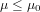# Hypothesis Testing

The objective of testing statistical significance of  (also ) by stating that we want to test the validity of the null hypothesis (HN), that the true population parameter β=0 against the alternative hypothesis (HA) that is different from zero (i.e. β=0).
In first hypothesis, we set
HN :β=0
HA: β≠0 (this is two-tailed test)
However, if we have prior knowledge about the sign of β (say positive) then the hypothesis set are as
HN :β =0
HA: β>0 (one tailed test)
In case of two tailed or one tailed test, the procedure to examine the statistical significance of , the decision rule is as follows:

• If |t*|> t∆ (n-2) i.e absolute value of the computed test statistic (t*) greater than the critical t at level of significance ∆ and degree of freedom n-2, then reject the HN and conclude is statistically significant at ∆ level of significance.

(where degree of freedom is the total number of observations less the number of independent constraints put on them. For k variable model, df= n-k, where n is the total number of observations)
The P- value Approach
Apart from providing computed t statistics for the estimated coefficients, also provide the p- values which can also be used as an alternative approach in assessing the significance of the estimated coefficients. P-value is the lowest level of significance at which the HN could be rejected based on the test statistic actually observed. For example, if p= 0.002, it implies that HN will be rejected at 2% level of significance. It is mandatory to reject the HN and conclude that the estimated coefficient under consideration is statistically significant if p-value is less or equal to 0.10.
Steps
1: State the Null Hypothesis.
2: State the Alternative Hypothesis.
3: Set α.
4: Collect Data.
5: Calculate a test statistic.
6: Construct Acceptance / Rejection regions.
7: Based on steps 5 and 6, draw a conclusion about H0.

Upper Tail Test of Population Mean with Known Variance

The null hypothesis of the upper tail test of the population mean can be expressed as follows:where μ0 is a hypothesized upper bound of the true population mean μ.

Let us define the test statistic z in terms of the sample mean, the sample size and the population standard deviation σ :Then the null hypothesis of the upper tail test is to be rejected if z zα , where zα is the 100(1α) percentile of the standard normal distribution.

Then the null hypothesis of the upper tail test is to be rejected if z zα , where zα is the 100(1α) percentile of the standard normal distribution.

#### Problem

Suppose the food label on a cookie bag states that there is at most 2 grams of saturated fat in a single cookie. In a sample of 35 cookies, it is found that the mean amount of saturated fat per cookie is 2.1 grams. Assume that the population standard deviation is 0.25 grams. At .05 significance level, can we reject the claim on food label?

#### Solution

The null hypothesis is that μ 2. We begin with computing the test statistic.

> xbar = 2.1            # sample mean
> mu0 = 2               # hypothesized value
> sigma = 0.25       # population standard deviation
> n = 35                  # sample size
> z = (xbarmu0)/(sigma/sqrt(n))
> z                             # test statistic
 2.3664

We then compute the critical value at .05 significance level.

> alpha = .05
> z.alpha = qnorm(1alpha)
> z.alpha                # critical value
 1.6449